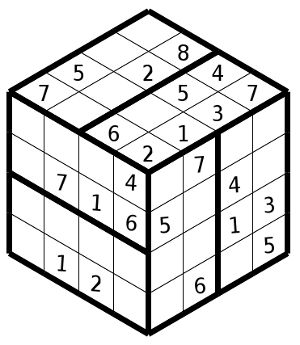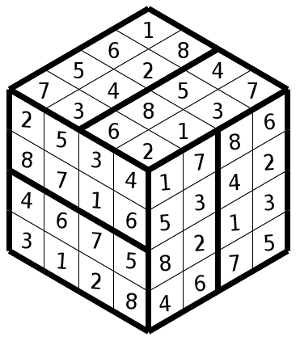3D Simple

## Rules of Sudoku 3D

For those who are bored with classic Sudoku and want new challenges.

## Sudoku 3D Simple

The grid of this Sudoku is in a shape of a cube, each row and column stretch on two faces of the cube. To follow a row or column, witout losing your way, you must start on an edge and go until the second parallel edge on the other side of the cube. Depending of the size of the puzzle, you have to use the numbers from 1 to 8 for a 4x4x4 cube, 1 to 12 for a 6x6x6 cube and finally 1 to 16 for the colossal 8X8X8 cube. Each numbers, as in the Sudoku, must appear once in each row, column and region. Usually the regions are in form of rectangle or square but for some puzzles the regions are in irregular shape.

This is a Sudoku 3D of size 4x4x4.Notice each row and column, on a given face, stretches on two faces of the cube, like the Sudoku, each region, row and column contains all the number from 1 to 8.

## Sudoku 3D with irregular regions.

This is a Sudoku 3D with irregular regions.Again you must put the number 1 to 8 in each column, row and region, except that regions are irregular.

You could find irregular Sudoku 3D of size 6X6X6 like this.

For this size you need the number 1 to 12 and each face contains 3 regions.

And finally the biggest Sudoku 3D with size 8X8X8.

You need the number 1 to 16 and each face contains 4 regions.

### Sudoku 3D Combined (Hyper Sudoku)

This is a combination of two or three Sudoku 3D binded together. For each cube, you use the rule of Sudoku 3D, but when a face of a cube touch a face from an another cube, this create a new row or column, formed by this two faces from two different cubes. This particularity could help you to resolve a cube by looking at the clues of the face of neighbouring cube. And you repeat this process until the resolution of the complete puzzle,

This is a hyper Sudoku with two cubes.

Notice the second face (at right) of the first cube. Rows start from the second face up to the first face and finish in the second face of this cube. But rows start form the second face of this first cube up to the first face of the second cube.  The hyper Sudoku could have irregular regions and also have cubes of size 6x6x6. In some Hyper Sudoku the cubes are placed in a shape of a pyramid like that: